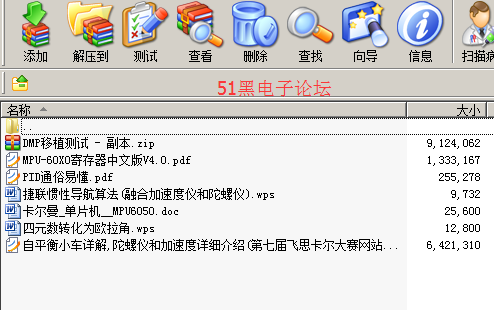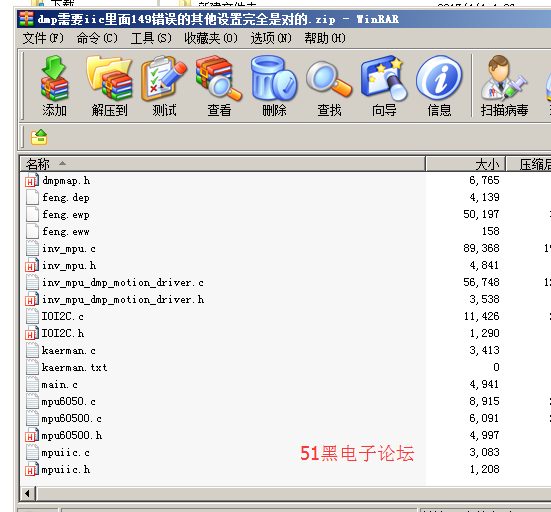##用户名 Email 自动登录 找回密码 密码 立即注册

# MPU6050 DMP直接输出姿态角，无需卡尔曼 MSP430主控[复制链接]51hei官方发布 发表于 2017-4-4 04:11 | 显示全部楼层 |阅读模式
 用了6050，我得说明几点，，一 ，关于姿态角解算，你可以卡尔曼，也可以直接算i四元数再结算，其中区别请看源程序， 二、dmp、dmp很简单、但是、需要具有iic的 接口 、能懂吗？ 需要有推挽输出能力的iic接口、不是模拟iic、我被这个气死了 ！！！！！！！！！！！！！！ 三、我懒得去整理  、直接打包上传的三个文件  有点乱、时间紧迫也没有去美化、你们可以看到我的这三种用法、如果自己用时哪里有问题  、请注意分析源代码 的计算原理、强调一点、iic 没成功是因为 149没有iic 、对于这个我也是日了狗、我的设置是全部可以参考的这个是别人的资料：平衡车全套资料.zip (15.25 MB, 下载次数: 111) 2017-4-4 04:05 上传 点击文件名下载附件mpu6050DMP.zip (1.24 MB, 下载次数: 76) 2017-4-4 04:04 上传 点击文件名下载附件这个是430的MPU6050-DMP.zip (1.33 MB, 下载次数: 55) 2017-4-4 04:05 上传 点击文件名下载附件MSP430单片机主程序： #include #include #include "Config.h" #include "1602.c" #include "mpu6050.c" #include "mpu60500.h" #include "mpu60500.c" #include "mpuiic.h" #include "mpuiic.c" #include "IOI2C.h" #include "IOI2C.c" #include "dmpKey.h" #include "dmpmap.h" #include "inv_mpu.h" #include "inv_mpu.c" #include "inv_mpu_dmp_motion_driver.h" #include "inv_mpu_dmp_motion_driver.c" #include "kaerman.c" struct quaternion{   float w;   float x;   float y;   float z; }quaternion; float gyr,acc; float Pitch=1.0,Roll,Yaw ; //q30格式,long转float时的除数. #define q30  1073741824.0f void mix_gyrAcc_crossMethod(struct quaternion *attitude,const float gyr,const float acc,float interval); void quaternion_normalize(struct quaternion*q); void main() {     WDT_Init();                         //看门狗设置   Clock_Init();                       //系统时钟设置   Port_init();                        //系统初始化，设置IO口属性   delay_ms(100);                      //延时100ms   LCD_init();                         //液晶参数初始化设置   LCD_clear();                        //清屏   //InitMPU6050();   uchar e=mpu_dmp_init();   float yy=e*1.0;   delay_ms(300);   /*   P1DIR|=0x01;          P1OUT&=0XFE;   TA0CCTL1=OUTMOD_7+CCIE;   TACTL=TASSEL_2+MC_1+TAIE;   TA0CCR0=655;   TA0CCR1=654;      */ //float aaa=0.0,bbb=0.0,ccc=0.0;   quaternion.w=1;   quaternion.x=0;   quaternion.y=0;   quaternion.z=0;         _EINT();   while(1)   {         Disp(yy,0,0);         /*     gyr=-GetData(GYRO_XOUT_H)/16.4;     gyr=-GetData(GYRO_YOUT_H)/16.4;     gyr=-GetData(GYRO_ZOUT_H)/16.4;     acc=GetData(ACCEL_XOUT_H)/16384;     acc=GetData(ACCEL_YOUT_H)/16384;     acc=GetData(ACCEL_ZOUT_H)/16384;         Disp(Pitch,0,0);     Disp(Roll,7,0);     Disp(Yaw,0,1);     Disp(quaternion.z,7,1);     mpu_dmp_get_data(&aaa,&bbb,&ccc);     Disp(aaa,0,0);     Display10BitData(GetData(ACCEL_XOUT_H),0,0);        //显示X轴加速度     Display10BitData(GetData(ACCEL_YOUT_H),5,0);        //显示Y轴加速度     Display10BitData(GetData(ACCEL_ZOUT_H),10,0);        //显示Z轴加速度     Display10BitData(GetData(GYRO_XOUT_H),0,1);                //显示X轴角速度     Display10BitData(GetData(GYRO_YOUT_H),5,1);                //显示Y轴角速度     Display10BitData(GetData(GYRO_ZOUT_H),10,1);                //显示Z轴角速度 */   } } /* #pragma vector=TIMERA1_VECTOR __interrupt void P1(void) {   P1OUT|=0X01;   if(TAIV==2)   {_NOP();}          mix_gyrAcc_crossMethod(&quaternion,gyr,acc,0.01); } */ void mix_gyrAcc_crossMethod(struct quaternion *attitude,const float gyr,const float acc,float interval) {   const static float FACTOR = 0.001;//取接近0的数   //   float w_q = attitude->w;   float x_q = attitude->x;   float y_q = attitude->y;   float z_q = attitude->z;   float x_q_2 = x_q * 2;   float y_q_2 = y_q * 2;   float z_q_2 = z_q * 2;   //   // 加速度计的读数，单位化。   float a_rsqrt = sqrt(acc*acc+acc*acc+acc*acc);   float x_aa = acc * a_rsqrt;   float y_aa = acc * a_rsqrt;   float z_aa = acc * a_rsqrt;   //加速度计测量出的加速度向量(载体坐标系下)   //   // 载体坐标下的重力加速度向量，单位化。   float x_ac = x_q*z_q_2 - w_q*y_q_2;   float y_ac = y_q*z_q_2 + w_q*x_q_2; //通过四元数旋转矩阵与地理坐标系下的重力加速度向量[0 0 0 1]叉乘得到载体坐标系下的重力加速度向量   float z_ac = 1 - x_q*x_q_2 - y_q*y_q_2;//（主要）角速度计测出的四元数表示的载体坐标系下的重力加速度向量（这里已转换成载体坐标系下）   //   // 测量值与常量的叉积。   float x_ca = y_aa * z_ac - z_aa * y_ac;   float y_ca = z_aa * x_ac - x_aa * z_ac;   float z_ca = x_aa * y_ac - y_aa * x_ac;//角速度计测出的角度误差，叠加的FACTOR大小可以实验试凑   //   // 构造增量旋转。   float delta_x = gyr * interval / 2 + x_ca * FACTOR;   float delta_y = gyr * interval / 2 + y_ca * FACTOR;   float delta_z = gyr * interval / 2 + z_ca * FACTOR;   //   // 融合，四元数乘法。   attitude->w = w_q         - x_q*delta_x - y_q*delta_y - z_q*delta_z;   attitude->x = w_q*delta_x + x_q         + y_q*delta_z - z_q*delta_y;   attitude->y = w_q*delta_y - x_q*delta_z + y_q         + z_q*delta_x;   attitude->z = w_q*delta_z + x_q*delta_y - y_q*delta_x + z_q;   quaternion_normalize(attitude);//归一化   float q0=0.0,q1=0.0,q2=0.0,q3=0.0;   q0=attitude->w;   q1=attitude->x;   q2=attitude->y;   q3=attitude->z;   Pitch = asin(-2 * q1 * q3 + 2 * q0* q2)* 57.3;        // pitch   Roll = atan2(2 * q2 * q3 + 2 * q0 * q1, -2 * q1 * q1 - 2 * q2* q2 + 1)* 57.3; // roll                    Yaw = atan2(2*(q1*q2 + q0*q3),q0*q0+q1*q1-q2*q2-q3*q3) * 57.3; } void quaternion_normalize(struct quaternion*q) {   float qlength_inv = 1.0/(sqrt(q->w*q->w + q->x*q->x + q->y*q->y + q->z*q->z));   //这里只应该开根号x*x+y*y+z*z   // now normalize   q->w=q->w*qlength_inv;     q->x=q->x*qlength_inv;   q->y=q->y*qlength_inv;   q->z=q->z*qlength_inv;    } 复制代码 自己做的程序：卡尔曼重要设置已保留.zip (3.17 KB, 下载次数: 25) 2017-4-4 04:06 上传 点击文件名下载附件四元数算.zip (493.84 KB, 下载次数: 34) 2017-4-4 04:06 上传 点击文件名下载附件dmp需要iic里面149错误的其他设置完全是对的.zip (143.94 KB, 下载次数: 21) 2017-4-4 04:06 上传 点击文件名下载附件white1994 发表于 2017-6-15 13:27 | 显示全部楼层
 厉害了，，，，些许冷热 发表于 2017-7-22 11:03 | 显示全部楼层
 贼强...............................insightx 发表于 2017-10-15 22:17 | 显示全部楼层
 正在研究了，借鉴一下。taohai163 发表于 2017-11-17 09:53 | 显示全部楼层
 我找了很久的资料，谢谢了hxybiseng 发表于 2018-4-25 10:40 | 显示全部楼层
 正好需要 下载看看  谢谢wenshajava 发表于 2018-4-25 17:31 | 显示全部楼层
 资料很好啊, 谢谢分享FangLilian 发表于 2018-5-11 09:39 | 显示全部楼层
 不好意思，本人小白，请问模拟IIC是什么啊jiangjxuan 发表于 2018-7-6 10:51 | 显示全部楼层
 怎么下载啊？爱生活！ 发表于 2018-7-19 15:08 | 显示全部楼层
 谢谢楼主分享mr_xcq 发表于 2018-9-20 20:08 | 显示全部楼层
 四元素算法，由于迭代导致欧拉角一直更新，换句话说欧拉角经过一定时间才会稳定，楼主是怎样使用的呢星的爱我 发表于 2019-3-8 22:12 | 显示全部楼层
 我也想知道四元数和卡尔曼滤波的问题。。。 还有那个iic,现在有点晕乎乎的Sakura666 发表于 2019-3-20 14:34 | 显示全部楼层
 谢谢楼主分享

 本版积分规则 回帖并转播 回帖后跳转到最后一页